# What is Nuclear Energy?

## What is Nuclear Energy?

Nuclear Energy:

1. In alpha and beta decay, energy is released in the form of kinetic energy of the daughter nuclide and radioactive emission. This is known as nuclear energy.
2. Nuclear energy comes from the loss of mass in a nuclear reaction.
3. Two nuclear reactions that release a large amount of energy are nuclear fission and nuclear fusion.

## What is nuclear fission and how does it occur?

Nuclear Fission

• In 1939, Otto Hahn discovered that a uranium-235 nucleus can capture a neutron that collides with it. This produces a compound uranium-236 nucleus which is highly unstable.
• The uranium-236 nucleus quickly splits into two lighter nuclei. At the same time two or three neutrons are produced.
• The process of splitting of a heavy nucleus into two lighter nuclei of comparable masses (after bombardment with a energetic particle) with liberation of energy is called nuclear fission.
• Figure gives a diagrammatic representation of nuclear fission.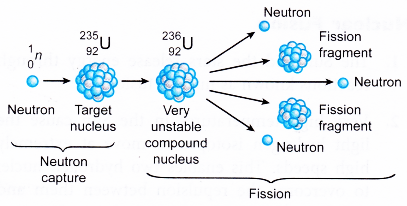• Fission reaction of U235
A possible equation for the fission of a uranium-235 nucleus can be written as follows:
$${}_{92}{{U}^{235}}+{}_{0}{{n}^{1}}\to {}_{92}{{U}^{236}}\to$$
( Unstable nucleus)
$${}_{56}B{{a}^{141}}+{}_{36}K{{r}^{92}}+3{}_{0}{{n}^{1}}+Q$$
• The energy released in U235 fission is about 200MeV or 0.8 MeV per nucleon.
• Fission of U235 occurs by slow neutrons only (of energy about 1eV) or even by thermal neutrons (of energy about 0.025 eV).
• The neutrons released during the fission process are called prompt neutrons.
• The energy is mainly in the form of kinetic energy of the fission fragments and electromagnetic radiation.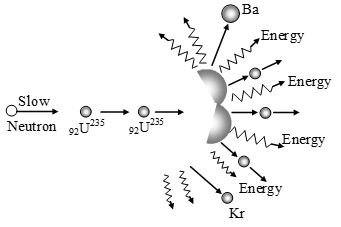• A nuclear fission reaction releases an enormous amount of energy. The energy released is a few hundred million times the energy released in an equivalent chemical reaction.
• There are a number of possible combinations of fission fragments. The heavier fission fragment usually has a nucleon number between 130 and 149. Some examples of fission fragments are: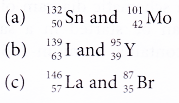• Another possible nuclear fission is:## How is energy released in a nuclear fusion reaction?

Nuclear Fusion:

• The Sun and the stars release energy through reactions known as nuclear fusion.
It has been observed that under special conditions, it is possible for the nuclei of light elements to combine and form a nucleus of a high atomic number. When two or more light nuclei moving at very high speed are fused together to form a heavy nucleus, then the process is known as nuclear fusion.
• Very high temperatures in the Sun cause the light hydrogen isotopes to move at extremely high speeds. This enables two hydrogen nuclei to overcome the repulsion between them and collide.
• When two hydrogen-2 (deuterium) nuclei collide, they can combine together to produce a heavier nucleus. This is known as a fusion reaction
• Nuclear fusion is the combining of two lighter nuclei to form a heavier nucleus.
• The mass of the product nucleus is smaller than the sum of the masses of the nuclei which were fused. The lost mass is converted into energy which is released in the process.
$$_{1}^{2}H\text{ }+\text{ }_{1}^{2}H\text{ }\to \text{ }_{1}^{3}H\text{ }+\text{ }_{1}^{1}H\text{ }+\text{ }4.0\text{ }MeV$$
Deuteron   Deuteron    Triton   Proton   Energy
• The triton so formed can further fuse with a third deuteron to form an α–particle (Helium–nucleus).
$$_{1}^{3}H\text{ }+\text{ }_{1}^{2}H\text{ }\to \text{ }_{2}^{4}H\text{ }+\text{ }_{0}^{1}\text{n }+\text{ 17}.6\text{ }MeV$$
• Figure shows a schematic diagram of a nuclear fusion reaction.• The following are more examples of nuclear fusion reactions:• A large amount of energy is released in nuclear fusion. The energy released in a fusion reaction is from the kinetic energy of the heavier nucleus and the energy of the neutron, proton or gamma rays. Energy released in nuclear fusion is very much more than in nuclear fission.
• Fusion can only occur when two nuclei are close enough for their nuclear forces to pull them together. Both the nuclei involved in a fusion reaction are positively-charged. They repel each other with increasing magnitude as they come closer together. This explains why fusion can only occur at very high temperatures. Fusion of hydrogen-2 nuclei requires a temperature of more than 50 million degrees Celsius.

## What is the difference between fission and fusion?

 S.No NUCLEAR FISSION NUCLEAR FUSION 1 A heavy nucleus  disintegrates to give lighter nuclei with the release of energy. Two or more light nuclei join together to form a heavy nucleus with the release of energy 2 Nuclear fission is a chain reaction-the fission of one nucleus leading to the production of neutrons, which cause more nuclei to disintegrate. Nuclear fusion is not a chain reaction. 3 Nuclear fission requires thermal neutrons neutronswith sufficient energy to split a nucleus. But it does not require very high temp. Nuclear fusion requires high temperatures in the range of 106K. 4 Fission process can be carried out in a reactor. Fusion cannot be carried out in any container, as the temperature is very high. Scientists hope to contain matter in a magnetic field 5 Controlled nuclear fission is possible and takes place in a nuclear reactor. Controlled nuclear fusion has so far not been achieved. Only uncontrolled fusion reactions in the thermonuclear devices such as the hydrogen bomb have been achieved. 6 Nuclear fission produces radioactive wastes, which pose the problem of waste disposal. Nuclear fusion does not produce radioactive wastes and, therefore, does not cause pollution.

## What happens in a nuclear chain reaction?

Chain Reactions:

• A nuclear fission reaction produces two or three free neutrons.
• These neutrons can strike other uranium nuclei and cause them to split to produce more fission fragments and more neutrons.
• A self-sustaining fission will occur if at least one of the neutrons split another uranium nucleus. This sets off a chain reaction in which nuclear fission spreads through a mass of uranium.
• A chain reaction is a self-sustaining reaction in which the products of the reaction can initiate another similar reaction.
• Figure shows a schematic diagram of how a chain reaction can be started in a sample of uranium that contains uranium-235 and uranium-238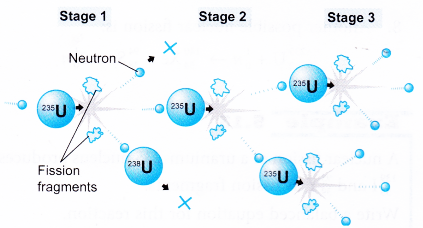(a) Stage 1
A uiamum-235 atom absorbs a neutron and fissions into two new atoms (fission fragments), releasing three new neutrons and energy.
(b) Stage 2
One of these neutrons is absorbed by an atom of uranium-238 and does not continue the reaction.Another neutron does not collide with anything and is lost. However, one neutron does collide with an atom of uranium-235 which then fissions and releases two neutrons and energy.
(c) Stage 3
Both of these neutrons collide with uranium-235 atoms, each of which fissions and releases between one and three neutrons which can then continue the reaction.
• A stable chain reaction occurs if on the average one neutron from each fission induces another fission.
• If more than one of the neutrons cause other fissions, the chain reaction occurs at an increasing rate.
• A controlled chain reaction is used in nuclear power stations while an uncontrolled chain reaction is used in nuclear bombs.
• The uranium sample must have a certain minimum mass to sustain a chain reaction. This is known as the critical mass.
• If the mass of the sample is larger than the critical mass, there will be sufficient uranium-235 nuclei to capture the neutrons.
• The neutrons produced in a fission reaction are very fast neutrons. The fast neutrons are more likely not to be captured by the uranium nuclei. The fast neutrons need to be slowed down so that they can be more easily captured by the uranium nuclei.
• Materials such as water and graphite can act as moderators to slow down the neutrons. This will enable a chain reaction to occur for a smaller critical mass.

### Energy in a Nuclear Reaction

• In both nuclear fission and fusion, the products of the reaction have a smaller mass than the mass before the reaction.
• The nuclear reaction results in a loss of mass known as mass defect.
• Consider a reaction represented by the equation:
V + W → X + Y
The mass defect, m is given by
m = (mv + mw) – (mx + my)
• According to Einstein, mass and energy are not conserved separately. In a nuclear reaction, mass and energy can be exchanged one for the other. Only the total “mass-energy” is conserved.
• A loss of mass in a nuclear reaction means that the mass had changed to energy. The relationship between mass and energy is given by the equation:
E = mc2
where, E = Energy released in joules (J)
m = Loss of mass or mass defect in kilograms (kg)
c = Speed of light = 3 × 108 m s-1

### Energy Released in Nuclear Fission

1. Consider the following fission of uranium-235.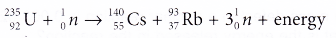Mass of a neutron = 1.008665 u
The atomic mass of uranium-235, caesium-140 and rubydium-93 are as follows:
uranium-235 = 235.043924 u
caesium-140 = 139.917277 u
rubidium-93 = 92.922033 u
Mass before fission,
mi = 235.043924 u + 1.008665 u
= 236.052589 u
Mass of the fission products,
mf = 139.917277 u + 92.922033 u + 3(1.008665 ) u
= 235.865305 uTherefore, the energy released by the fission of one uranium-235 nucleus is 2.80 × 10-11 J.
2. The amount of energy released by the splitting of one nucleus is more than a million times larger than the energy released per atom in a chemical reaction.

### Energy Released in Nuclear Fusion

1. Consider the following nuclear fusion:2. This amount of energy is also very large compared to the energy released per atom in a chemical reaction.

### Energy Released in an Alpha Decay

1. Einsteins equation is also used to calculate the energy released in alpha and beta decay.
2. The most abundant naturally-occurring isotope of uranium is uranium-238. It undergoes alpha decay to thorium-234. The decay is represented by the equation:The values of their atomic masses are as follows:
Mass of uranium-238 = 238.050785 u
Mass of thorium-234 = 234.036596 u
Mass of helium-4 = 4.002603 u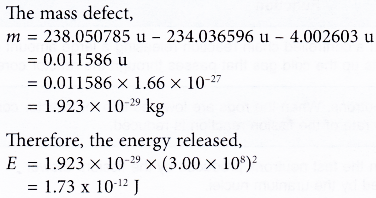3. The energy released is mainly in the form of the kinetic energy of the thorium nucleus and the a-particle.

### Energy Released in a Beta Decay

1. Consider the decay of carbon-14, given by the equation: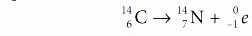Mass of carbon-14 = 14.003242 u
Mass of nitrogen-14 = 14.003074 u
2. The mass of carbon-14 includes the mass of 6 electrons and the mass of nitrogen-14 includes the mass of 7 electrons. However, the nitrogen atom created by the decay contains only 6 electrons.
3. Therefore, when calculating the mass defect, it is not necessary to include the mass of the β-particle because it is already included in the mass of the nitrogen-14 atom.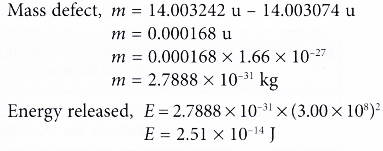### Destructive Form Of Nuclear Energy

(A) Nuclear Bomb
This produces tremendous amount of destructive energy. This energy is produced by uncontrolled nuclear fission chain reaction.
The atom bomb or nuclear bomb produces large amount of energy when two pieces of uranium (U235) or Plutonium (Pu239) are brought in contact so that the total mass become greater then critical mass.
During this process a very high temperature of the order of million degree kelvin is produced and also a very high pressure of the order of several million atmosphere is developed.
(B) Hydrogen Bomb
It produces extremely high destructive energy by using nuclear fusion. The fusion reaction is performed over heavy–hydrogen nuclei at very high temperature and pressure.
To get this high temperature and pressure, nuclear fission bomb is used at the centre core of a hydrogen bomb which is formed of heavy hydrogen like lithium hydride (LiH2).